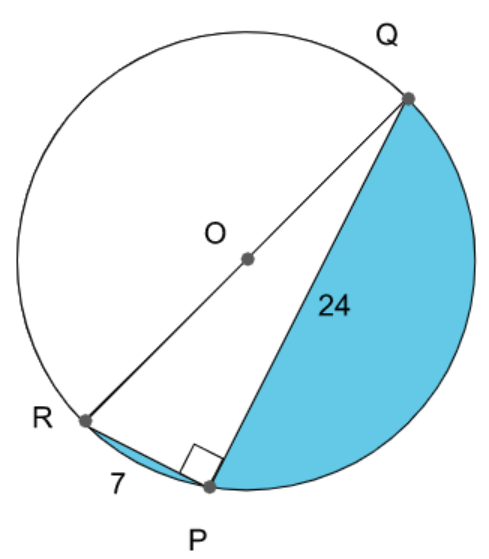QuestionAnswers

# Find the area of the shaded region in the figure, if PQ=24cm, PR=7cm and O is the center of the circle.A. $101.98c{{m}^{2}}$B. $161.54c{{m}^{2}}$C. $101.54c{{m}^{2}}$D. None of theseVerified
130.2k+ views
Hint: In the problem we need to find the shaded region so what we have to do is finding the area of right angled triangle in semicircle part by using the formula for area of triangle and then we have to find the area of semicircle. Now by subtracting the area of the right angled triangle from the area of the semicircle we will get the area of the shaded part.

We know that any angle made by the diameter QR in the semicircle is ${{90}^{\circ }}$
Hence $\angle RPQ={{90}^{\circ }}$
In the right angled $\Delta RPQ$
By Pythagoras we know that
$R{{Q}^{2}}=P{{Q}^{2}}+P{{R}^{2}}$
\begin{align} & R{{Q}^{2}}={{24}^{2}}+{{7}^{2}} \\ & R{{Q}^{2}}=576+49 \\ & R{{Q}^{2}}=625 \\ & RQ=\sqrt{625} \\ & RQ=25cm \\ \end{align}
We know that RQ is the diameter of circle so the radius of circle is $OQ=\dfrac{RQ}{2}=\dfrac{25}{2}cm$
The area of right angled $\Delta RPQ=\dfrac{1}{2}\times base\times height$
The area of right angled $\Delta RPQ=\dfrac{1}{2}\times RP\times PQ$
The area of right angled $\Delta RPQ=\dfrac{1}{2}\times 7\times 24$ $=7\times 12=84c{{m}^{2}}$. . . . . . . . (1)
We know that the area of semicircle is given by the formula $=\dfrac{\pi {{r}^{2}}}{2}$
$=\dfrac{22}{7}\times \dfrac{25}{2}\times \dfrac{25}{2}\times \dfrac{1}{2}$
$=\dfrac{11\times 25\times 25}{28}$
$=\dfrac{6875}{28}c{{m}^{2}}$. . . . . . . . . . . (2)
The area of shaded region is given by area of semicircle -area of right angled $\Delta RPQ$
$=\dfrac{6875}{28}-84$
$=\dfrac{6875-2352}{28}$
$=\dfrac{4523}{28}=161.54c{{m}^{2}}$
Hence the area of the shaded region is $161.54c{{m}^{2}}$

So, the correct answer is “Option B”.

Note: we know that the formula for area of triangle is given by $\dfrac{1}{2}\times base\times height$ and formula for area of semicircle is given by $\dfrac{\pi {{r}^{2}}}{2}$. The diameter of the circle cuts the given circle into two equal parts in other words it divides into two semicircles. In this problem line RQ divides into two semicircles.